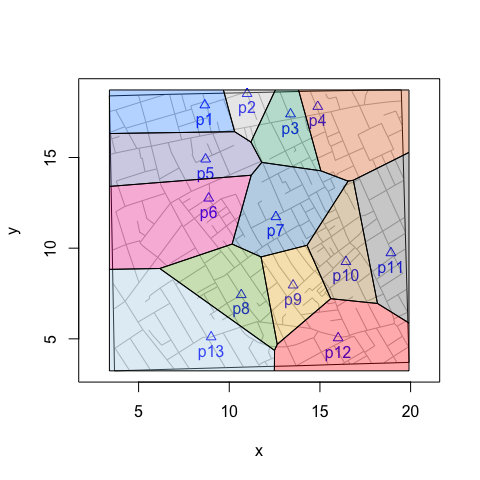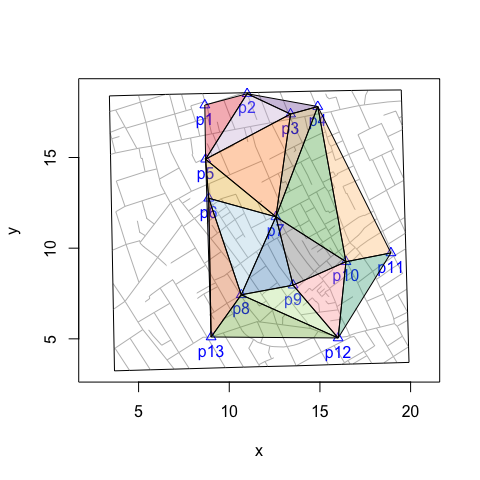# voronoiPolygons(): Tiles, Triangles and Polygons

#### 2021-04-21

voronoiPolygons() is a wrapper function that extracts the vertices of ‘deldir’ Delauny triangles and Dirichelet (Voronoi) tiles for use with functions like graphics::polygon(). The function returns a list of data frames of vertices. This makes tasks like coloring tiles or triangles or counting cases within those tiles or triangles easier.

voronoiPolygons(sites, rw.data = NULL, rw = NULL, type = "tiles")

The functions has four arguments. sites is the data frame of the sites or focal points used to do the tessellation or triangulation. rw.data (rw = ‘rectangular window’) is the data frame of a secondary source data (e.g., fatalities, customers, etc.). This argument is useful when the range of secondary data exceeds that of the sites data. rw is the deldir way to specify the range of data. It uses a vector of the corners of the rectangular window: xmin, xmax, ymin, ymax. type is “tiles” or “triangles”.

To color tiles and triangles or to count the number of points (e.g., fatalities) within each tile or triangle, we can apply sp::point.in.polygon() to the results of voronoiPolygons().

## Count points within tiles

# compute vertices of Voronoi tiles
vertices <- voronoiPolygons(sites = cholera::pumps, rw.data = cholera::roads)

# locations of the 578 fatalities in Soho
cases <- cholera::fatalities.unstacked

# count fatalities within each tile
census <- lapply(vertices, function(tile) {
sp::point.in.polygon(cases$x, cases$y, tile$x, tile$y)
})

# ID the 13 water pumps
names(census) <- paste0("p", cholera::pumps$id) # count of fatalities by neighborhood vapply(census, sum, integer(1L)) > p1 p2 p3 p4 p5 p6 p7 p8 p9 p10 p11 p12 p13 > 0 1 13 23 6 61 361 16 27 62 2 2 4 ## Count points within triangles # compute vertices of Delauny triangles vertices <- voronoiPolygons(sites = cholera::pumps, rw.data = cholera::roads, type = "triangles") # locations of the 578 fatalities in Soho cases <- cholera::fatalities.unstacked # count fatalities within each triangle census <- lapply(vertices, function(tile) { sp::point.in.polygon(cases$x, cases$y, tile$x, tile\$y)
})

# ID triangles
names(census) <- paste0("t", seq_along(vertices))

# count of fatalities by triangle
vapply(census, sum, integer(1L))
>  t1  t2  t3  t4  t5  t6  t7  t8  t9 t10 t11 t12 t13 t14 t15 t16 t17
>   1   0   1  11  43 179  35   2  18 138  15  22  97   0   0   4   1

## Color tiles

# compute vertices of Voronoi tiles
vertices <- voronoiPolygons(sites = cholera::pumps, rw.data = cholera::roads)

# define colors
snow.colors <- grDevices::adjustcolor(snowColors(), alpha.f = 1/3)

# plot map and color coded tiles
snowMap(add.cases = FALSE)
invisible(lapply(seq_along(vertices), function(i) {
polygon(vertices[[i]], col = snow.colors[[i]])
}))## Color triangles

# compute vertices of Delauny triangles
vertices <- voronoiPolygons(sites = cholera::pumps,
rw.data = cholera::roads, type = "triangles")

# define colors
colors.pair <- RColorBrewer::brewer.pal(10, "Paired")
colors.dark <- RColorBrewer::brewer.pal(8, "Dark2")
brewer.colors <- sample(c(colors.pair, colors.dark))
colors <- grDevices::adjustcolor(brewer.colors, alpha.f = 1/3)

# plot map and color coded triangles
snowMap(add.cases = FALSE)
invisible(lapply(seq_along(vertices), function(i) {
polygon(vertices[[i]], col = colors[[i]])
}))